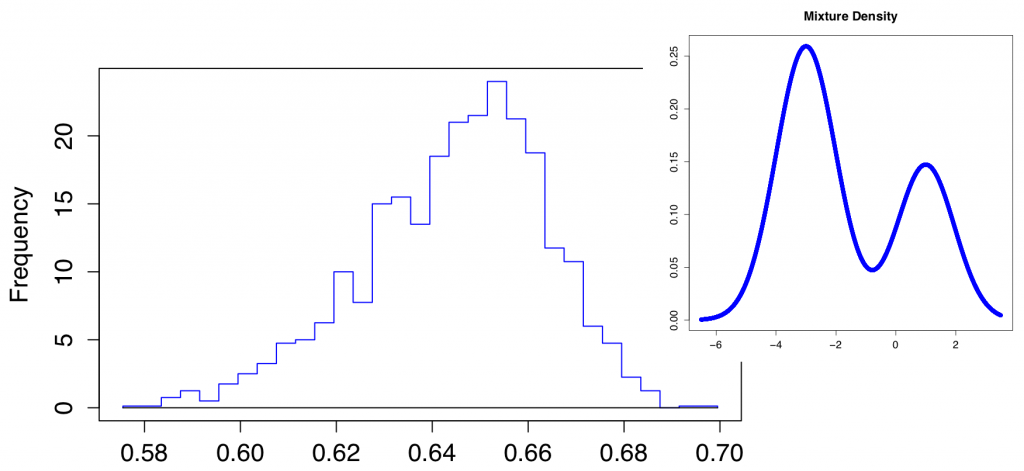# Focus on a joint research project: GOALGOAL (2015-2017) Geometry and Optimization with ALgebraic methods

#### Principal Investigators:

• Jean-charles Faugere, POLSYS project team, Inria Paris
• Bernd Sturmfels, U.C. Berkeley

#### Research objectives:

GOAL develops algorithms and mathematical tools to solve geometric and optimization problems through algebraic techniques. The long-term objective is to develop new software to solve more efficiently these problems. These objectives encompass: identifying instances of these problems that can be solved in polynomial time with respect to the number of solutions, and how to model these problems with polynomial equations.#### Scientific achievements:

• Algebraic Statistic. The goal is to recover all the parameters of a n-dimensional Gaussian from the set of vectors of moments of order ≤ d. This problem can be reduced to solve a polynomial system of equations. We investigate this fundamental problem in many directions:
• Computer Algebra: Since the polynomial systems are very hard to solve, we need to use as much as possible the symmetries of the problem. For instance to recover the parameters of a mixture of 3 Gaussians in dimension 1 the corresponding algebraic system has 225 complex solutions. In the case of a mixture of 2 Gaussians, using the symmetries we can easily recover the identifiability result of the system (Pearson) and the uniqueness result (Lazard).
• Geometry. From a geometrical point of view, we study the moment variety whose points are the vectors of all moments up to some order of a family of probability distributions. Following up on Pearson’s classical work from 1894, we apply current tools from computational algebra to recover the parameters from the moments. Our moment varieties extend objects familiar to algebraic geometers. For instance, the secant varieties of Veronese varieties are the loci obtained by setting all covariance matrices to zero. We compute the ideals of the 5-dimensional moment varieties representing mixtures of two univariate Gaussians, and we offer a comparison to the maximum likelihood approach.
• Multi view Geometry Structured polynomial systems coming from matricial problems arise also naturally in multi-view geometry. Recently, epipolar geometry (which consists in studying image reconstruction from 2 views) has attracted some attention on the algebraic side. We have studied a real root classification problem, which consists in identifying the positions of the cameras for which there is a unique possible image reconstruction. This problem is highly challenging from a computational viewpoint: the polynomial system involves 14 parameters and has large degree. The complete classification has been computed and a report on these results is under progress.

We propose new software to solve this problems more efficiently.

Other Activities: we organized two international workshops:

• International Workshop in Berkeley – May 17-19 2015
• International Workshop in Paris – May 20-21 2016

#### Publications and Awards:

• K. Kubjas has been awarded a Marie Curie grant (between MIT and Sorbonne Université/Inria).

#### Selected Publication:

• C. Amendola, J. C. Faugere, and B. Sturmfels. Moment varieties of Gaussian mixtures. Journal of Algebraic Statistics, 7:14-28, 2016.